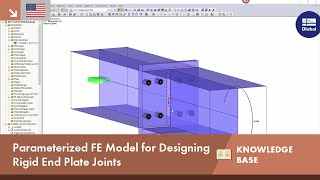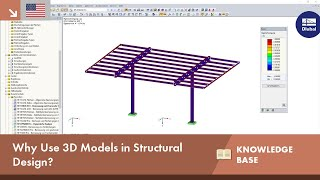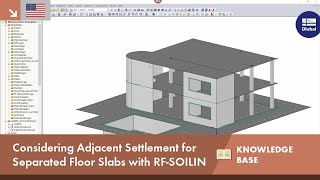# Equation solving methods for nonlinear calculations

### Technical Article

New

30 October 2020

001662

This article explains the equation solver for a nonlinear calculation with a Newton-Raphson iteration.

#### Introduction

The finite element method is always used when mechanical problems cannot be solved analytically. Often, nonlinear effects such as failure under compression (geometric nonlinearity), plastification (material nonlinearity), and contact or kinematic degrees of freedom are also taken into account. These effects, especially in the case of nonlinear material models, can be taken into account by means of an iterative calculation method.

#### FEM formulation

Basic steps of an FEM formulation (further information can be found in  ):

1. Weak form of equilibrium

Weak Form

$$∫Bgradδu⋯σdV⏟nichtlinearer Anteil = ∫Bδu · ρbdV + ∫∂Bδu · todA$$

 δu Virtual (test) displacement t0 Initial load factor σdV Internal forces ρbdV Solid forces B Integrated area
2. Converting to Voigt Notation with Tensor 4. Level

Weak Form in Voigt Notation

$$∫Bδε⋯ℂ⋯εdV = ∫BδuTbdV⏟Volumenkräfte + ∫∂BδuT · t0dA⏟Oberflächenkräfte$$

 C Stiffness matrix δε Variation of the strain state B Integrated area ε Strain U Deformation

This notation is used to solve the approximate solution of the FE approach for nonlinear materials.
3. For this, the displacement field is multiplied by the application functions.

Displacement Field via Trial Function

$$u(x,t) = H(x)u^(t)$$

 u(x,t) Displacement over time (load increment) H Form function û Nodal displacement
4. Using the derivative of the displacement in the weak form. Numerical integration is used to calculate the nodal displacement, and in post-processing, the stresses and strains are calculated using the material law.

#### Sequence of a Newton-Raphson iteration

Due to the nonlinear material behavior, the material matrix C in Equation 2 above changes with each strain step. The standard calculation method to solve this problem is the so-called Newton-Raphson iteration. It is used to linearize the function in a starting point. In the iteration, the stiffness matrix C of the step is always used. In the linearized iteration step, a tangent is placed at the zero point of the function.

The equations belonging to the flow diagram in the figure above:

$$fextt + ∆t = fextt + ∆f$$

2. Predictor step

Predictor Step

$$K0t∆0φ = fextt+∆t - fint0t$$

 K Stiffness matrix of the previous time step t+Δtfext External forces increased by a further load step 0tfint Internal forces of the previous time step ϕ Strain
3. In point 3 Iteration of the flow diagram, the total distortion minus the plastic distortion is calculated (corrector step).

The goal of the iterative calculation is always that the sum of the loads results in zero. However, this is not possible numerically. Therefore, an abort rate ε is defined at which the calculation is aborted with sufficient accuracy.

Abortion Rate

$$R = fextt + ∆t - fintnt + ∆t < ε$$

 R Abortion rate fext External forces fint internal forces ε Epsilon abortion rate t Time step

In the program, you can control the termination rate under the calculation parameters.

The following figure shows the process of a Newton-Raphson iteration. In the first iteration

1. Iteration

$$Rt + ∆t - F0t + ∆t$$

 R Abortion rate t Time step F Force

the abort rate R or ε is not reached. Also in the second iteration (red), the tolerance limit is not reached. Only in the third iteration is the distance of the tangent stiffness so small that convergence is achieved.

As already mentioned, the deformation is continuously added up during the iteration.

#### Conclusion

The Newton-Raphson iteration has the consistency or convergence order 2. The number of "correct" locations of the iteration doubles for each step. As a result, a Newton-Raphson iteration converges quadratically and the accuracy increases with each iteration as the method converges. However, if the method does not converge, the error goes to infinity and the calculation is aborted.

The causes of the error are, for example, a too steep slope of the load-deformation curve and a too flat slope of the curve in the plastic area. If the load-deformation curve in the figure above shows a too strong buckling in the second iteration step, the material tangent and thus the stiffness matrix would be the slope of the elastic range. In this case, the slope of the zero point would be incorrect for the plastic area. This is one of the reasons why increasing the load steps is accompanied by improved convergence.

#### Reference

  Nackenhorst, U.: Vorlesungsskript Numerische Mechanik. Hannover: Institut für Baumechanik und Numerische Mechanik, Gottfried Wilhelm Leibniz Universität, 2013

### Write Comment...

• Views 454x
• Updated 21 November 2020Do you have questions or need advice?
Contact our free e-mail, chat, or forum support or find various suggested solutions and useful tips on our FAQ page.## KB 001678 | Parameterized FE model for design of rigid end plate joints

Length 0:59 min## KB 001680 | Why 3D Models in Structural Design?

Length 0:21 min## KB 001654 | Consider Adjacent Settlement for Separated Floor Slabs With RF-SOILIN

Length min## KB 001675 | Timber Column Design per the CSA O86-19 Standard

Length 1:55 minLength 2:03 min